# AI Starter- Build your first Convolution neural network in Keras from scratch to perform…

Source: Deep Learning on Medium

Hello there,

How are you doing? Welcome to the AI Starter series part -3.

I hope you have read part 1 and 2 of this series where I have explained the basics of machine learning, deep learning framework, introduction to Keras code syntax, trained and tested a simple neural network using fully connected layers.

In this blog, we will learn to build a multi-class classifier model using convolution layers. We will build a model to classify a test image into any of these three classes, panda, dog or cat. The objective will also be to increase the accuracy, decrease the loss and the miss-classification count during prediction as compared to the previous part -2 of the AI Starter series where we had built the model using dense or fully connected layers.

In convolutional neural networks (CNN) every convolution network layer acts as a detection and learning filter for the presence of specific features or patterns extracted from the training data and as these filters convolve with a huge number of training images they detect and simultaneously learn those features from the training images. For example in an image of a human face, its lips, eyes, nose, eyebrows, face shape, skin color and more could be a feature.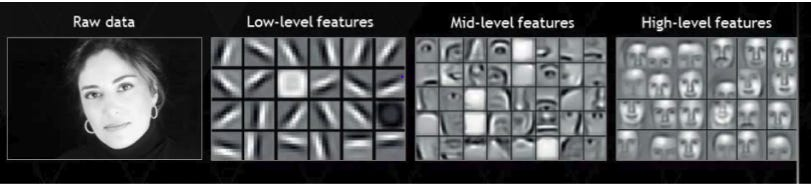As we go deeper into the network from convolution layer 1 to the last fully connected layer, more abstract features are learned. In the above image, the low-level features were extracted at the initial convolution layers of the CNN network. These features are small curves, lines and as we move from layer 1 to the intermediate layers of the network mid-level features like a nose, an eye, lips are learned, the last layers of the network extracts and learns features of a full human face.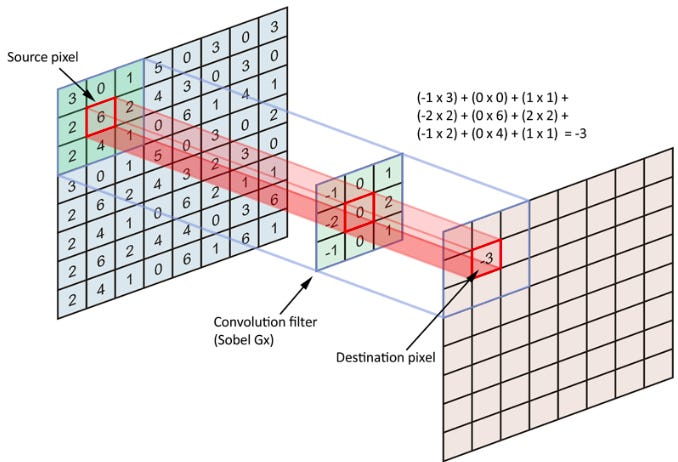There are three matrices in the above image. Left most matrix is the input image, the middle matrix is a filter which extracts and learns the features from the input image, the rightmost matrix is the output matrix =input image matrix convolved with the filter matrix. An 8×8 image is convolved with a 3×3 filter to produce a 7×7 feature map. The features from the images are extracted during forward propagation. The value of the filters is updated/learned during backward propagation.

Unlike convolution, In a fully connected layer, every neuron is connected to every neuron in the next layer, and each connection has its own weight. It’s also very expensive in terms of memory (connection between two neurons has a weight, which would certainly be large in number for the whole network) and computation (a very large number of connections among the neurons). Having being told that let us learn by doing (build the model) to see how convolution neural networks perform better than neural networks build only with fully connected layers.

#### Project structure

`|------AI_STARTER_CNN_CLASSIFIER| |------data| | |------animals| | | | |------|dogs| | | | |------|cats| | | | |------|pands| |------code| | |------train.py| | |------predict.py| |------test_images| | |------dogs.jpg| | |------cats.jpg| | |------pandas.jpg| |------output| | |------smallvggnet.model.pickle| | |------smallvggnet.model.model | | |------training_performance.png`

The data folder has three folders dogs, cats, and pandas. Each folder has 1000 images. We will split these images into train and test later in the code.

In the code folder, you can find two code files train and predict. The file train.py has the model and we use this code file to train our model. The file predict.py will use the learning to predict results on test images.

The output folder has a pickle file which is a serialized label binarizer file. This file contains an object which contains class names. It accompanies a model file. The model file is a serialized Keras model file is generated after training and can be used in future inference scripts. The training performance file will have a performance plot of training/validation of the training process for every epoch.

Always remember to follow Keras 7 steps to build a Deep learning model.

`1. Analyze the dataset2. Prepare the dataset3. Create the model4. Compile the model5. Fit the model6. Evaluate the model7. Summary`

#### Build your first CNN model

We already have the dataset with us for three classes dog, cats, pandas. The dataset is analyzed with care so that you can train and test on your CPU. You can add more images to it later if you wish to train the model on a GPU.

So let us start building our model. You can create a file with name train.py and start building the model.

Each part is explained with a block of code. The code is commented well. For a block of code line number and its explanation is with respect to that code block.

The model that we are about to build can be called as smallVGG Network which is derived from the very famous VGG16 architecture which contains 16 weight layers (13 convolutional layers and 3 fully connected layers). They are called as weight layers because in these layers parameters or weights are learned. The smaller version of VGG16 is made so that you can train and tets the network on your CPU and see a good result, unlike VGG16 which requires huge processing power (GPU). SmallerVGG has 6 convolution layer and 2 Dense layers.

Step -1 Import all the packages

• matplotlib: This is the go-to plotting package for Python. That said, it does have its nuances, and if you’re having trouble with it, refer to this blog post. On Line 3, we instruct matplotlib to use the “Agg” backend enabling us to save plots to disk.
• sklearn: The scikit-learn library will help us with binarizing our labels, splitting data for training/testing, and generating a training report.
• Keras: It is a deep learning framework. keras.models — There are two types of models in Keras: the Sequential model, and the functional model. The difference between sequential and functional model is that in the sequential model output of one layer can go only into the very next layer to it but in the functional model the output of any layer can follow any sequence of flow of data from one layer to another. keras.layers has many types of layers like Conv2D, MaxPooling2D, Activation, Dropout, Flatten, Dense. All of these layers have mathematical equations running in their backend to extract features, learn features, optimize learning parameters. One that we are using is the dense layer (fully connected layer). keras.optimizers provide us many optimizers like the one we are using in this tutorial SGD(Stochastic gradient descent). Keras.backend helps you to define the input data format according to the backend you are using. We are using tensorflow as the backend.
• imutils: pyimagesearch convenience functions. We’ll use the paths module to generate a list of image file paths for training.
• numpy: NumPy is for numerical processing with Python. It is another go-to package.
• pickle: It is used for serializing and de-serializing a Python object structure. Any object in python can be pickled so that it can be saved on disk. What pickle does is that it “serializes” the object first before writing it to file. Pickling is a way to convert a python object (list, dict, etc.) into a character stream. The idea is that this character stream contains all the information necessary to reconstruct the object in another python script. Pickle has two main methods. The first one is the dump, which dumps an object to a file object and the second one is load, which loads an object from a file object.
• cv2: This is OpenCV. Open Source Computer Vision Library.

…the remaining imports are built into your installation of Python!

#### Step -2 Load the image data from the disk

After you have imported all the dependencies, let us load the 3000 images into a numpy array data, of size (3000,12288), where rows = 3000 and columns = 12288 (image_height*image_width*number of channels =12288)(64*64*3 = 12288)and the labels of images into a numpy array labels of size (3000,), where rows = 1 and columns = 3000.

Line 3 and 4 –Initialize two empty list data and label. The data list will later store all the 3000 images. The label list will save all the labels corresponding to 3000 images.

Line 6- Give the path of the folder “animal” which has three sub-folders cats, dogs, pandas. Each folder has 1000 images.

Line 9-Creates a list of 3000 image path. It creates a single list by of absolute path of images by concatenating the file name of each 3000 images with its respective folder name.

Line 11 to 13 –Counts the total number of available images. This gives an idea to decide on how many images out of available images would you want to use for training and testing.

Line 14-Very important line. It helps you to randomly shuffle the images name in the image path list. This helps you to train the model equally for all the classes and not to follow a particular sequence of training(dog images first, then cats and then pandas). Shuffle is a very important step.

Line 17-Loop over all the shuffled image path list. Helps to load image data and labels one by one.

Line 21-Read images one at a time using OpenCV function imread. The image height, width can vary but the channels will remain the same. The number of channels will always be 3. OpenCV stacks channels in Blue, green, red (BGR) format.

Line 22 –We know that the images are of different shapes. Let us resize all the images to a uniform shape of height = 64 and width = 64 and channel =3. This will help us to fix an input size to our deep learning model. For resizing the image we use an OpenCV function resize. The total number of pixels in a single image would be 64x64x3 = 12288. You might wonder what can we learn from a 64x64x3 image. Yes, you are correct learning from such a small image is difficult. But using a bigger image will cost us for high computation power. But right now to train on CPU, test and understand the concepts we must keep going.

Line 36-Here we create a list of numpy arrays. Each numpy array is the image data of shape(64x64x3). The list “data” has 3000 numpy arrays.

Line 27,28-Line 27 extracts the class/label of an image from the filename of the corresponding image. If you open the dog’s folder you will find the naming convention is “dogs_number.jpg”. Here the “dog” in the filename is the class/label/ground truth of the image which helps us to know which class does the image belong to. In simple language which animal is there in the image. Line 27 appends the label into a list.

Line 32- Line 32 scales the value of pixel intensities between lower limit 0 and upper limit 255. This is a data pre-processing step. The image is an 8-bit image. The largest value of pixel intensity could be 255. So, we divide each number by 255 to normalize the data. Then we convert the list into a numpy array. The size of this numpy array would be (3000, 64,64,3). In (3000, 64,64,3) I think by now you have an idea that 3000 stands for the number of total images and image_height = 64, image_width =64, number_of_channels =3.

Line 33-Converts the labels list to a numpy array of shape (3000,). Rows = 1, Columns = 3000.For example — Label corresponds to the true value(class or label) of the image data data. Label corresponds to the true value of the image data data. Label corresponds to the true value of the image data data.

Step -3 Creating training, testing data split and augmentation

Line 3 and 4-We know that the total number of images is 3000. We have also loaded the data of 3000 images into a numpy array. We now must decide how many images out of 3000 will be used for training and how many images we will use for testing. Scikit learn helps us do this with a function train_test_split(). We just need to input our numpy array data and labels of 3000 images and specify the percentage of images we want to use as testing data. In the code, we have defined that we have defined the percentage, test_size = 0.25 which means that we want 25 percent of the image to be treated as test images. You can see below the split and the image count for train and test images.

`Number of training images = 2250 ,Number of training labels = 2250Number of testing images = 750 , Number of testing labels = 750`

Line 6 to 9-I would recommend you to first finish reading the article and go through my code for a binary classification to understand how to_catagorical function works.

Line 10, 11 and 12- Label binarization takes place in all of these lines of code. Before this line type of the labels is a string. Now we need to encode it to binary. So, to binarize the labels we use the Scikit learn label binarizer. This binarizer is present in the preprocessing module of Scikit learn. lb = preprocessing.LabelBinarizer(). One-hot encoding is performed on these labels making each label represented as a vector.

`[1, 0, 0] # corresponds to cats`
`[0, 1, 0] # corresponds to dogs`
`[0, 0, 1] # corresponds to panda`

A call to fit_transform finds all unique class labels in trainY and then transforms them into one-hot encoded labels.

A call to just .transform on testY performs just the one-hot encoding step — the unique set of possible class labels was already determined by the call to .fit_transform

Line 15- In Keras, keras.preprocessing.image.ImageDataGenerator() does real-time data augmentation. All deep learning problem needs data, lots and lots of data. ImageDataGenerator(), helps you to generate variations of images on the go while your training is going on. You might ask me a question of how does the system understands what kind of images must be generated during augmentation.

So, the answer is, there are two ways, the first one is fit(x, augment=False, rounds=1, seed=None), where x is some sample data. This computes the internal data stats related to the data-dependent transformations, based on an array of sample data. The second way is to specify the augmentation parameters on your own. We will see the second option in the code.

The second method needs all your creativity, patience, analytical skills.

We already have a dataset, but we wish to bring more variations into it thereby increasing the image count, which also means augmenting.

`rotation_range=30,width_shift_range=0.1,height_shift_range=0.1,shear_range=0.2,zoom_range=0.2,horizontal_flip=True,fill_mode="nearest"`

Below are some tips for getting the most from image data preparation and augmentation for deep learning.

• Review Dataset. Take some time to review your dataset in great detail. Look at the images. Take note of image preparation and augmentations that might benefit the training process of your model, such as the need to handle different shifts, rotations or flips of objects in the scene.
• Review Augmentations. Review sample images after the augmentation have been performed. It is one thing to intellectually know what image transforms you are using, it is a very different thing to look at examples. Review images both with individual augmentations you are using as well as the full set of augmentations you plan to use. You may see ways to simplify or further enhance your model training process.
• Evaluate a Suite of Transforms. Try more than one image data preparation and augmentation scheme. Often you can be surprised by the results of a data preparation scheme you did not think would be beneficial.

Line 19 to 23– Define the shape of the input image in the form of a tuple.

Line 25-Gives us the names of classes that we are going to train for. Here it would give us -dog, cat, and pandas.

Step — 4 Create your Keras CNN model

Let us start to build our model for the multiclass classification.

Type of the model that we are building is sequential. The output of layer 1 can go only go into layer 2 of the model and the output of layer 2 to layer 3 only. The output of layer 1 can never go into layer 3. No layer can be skipped.

Line 3 to 8– I want you to pay high attention to these 5 lines of code. If you can understand this you can understand the rest of the code in a few minutes because the same block of code is repeated multiple times. So, this block of code from line 3 to 8 has five layers as stated below:

`model.add(Conv2D(32, (3, 3), padding="same",input_shape=inputShape))model.add(BatchNormalization())model.add(Activation("relu"))model.add(MaxPooling2D(pool_size=(2, 2)))model.add(Dropout(0.25))`

Conv2D, the name says it all, Conv2D is a two-dimensional convolutional layer which performs convolution between two 2D matrices. In our case this convolution happens between an input image of size(height= 64, width=64, channels = 3) with 32 unique filters each of size (height=3, width=3).

The value of these filters is initialized by Keras. Keras does Xavier initialization to initialize the values of the filters. The values of filters are known as learned parameters or weights. Keras gives you the flexibility to choose the way you wish to initialize the filter values. But picking the right way of initialization needs a good study.

The second argument is padding. Padding could have two values “same” or “valid”.

When stride is 1 we can think of the following distinction:

• “same”: output size is the same as input size. This requires the filter window to slip outside the input map, hence the need to pad.
• “value”: Filter window stays at a valid position inside the input map, so output size shrinks by (filters_size-1). No padding occurs.

Padding the image will save us from shrinking outputs and loosing information on the corners of the image.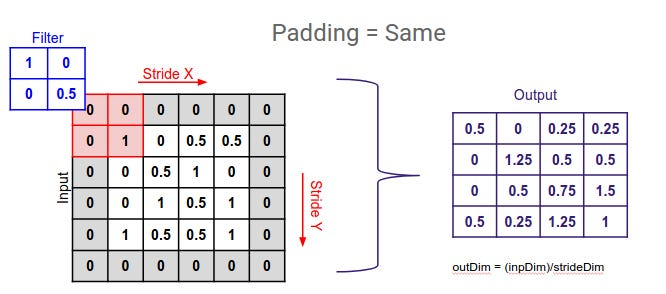In the above image, the input image is of shape 4X4(consider only the white colored matrix of the leftmost input matrix), Size of the filter is 2X2, Output feature map size is the same as the input image (4X4). To make the size of input and output equal we padded the input image. The gray colored zero borders you see in the leftmost matrix which has converted the input matrix of size 4X4 to 5X5 is called padding. Padding helps to preserve the information from the corners of the image.

Batch normalization is a technique to provide any layer in a Neural Network with inputs with zero mean, unit variance or any other mean and variance which the network forces to. To improve the training, we seek to reduce the internal covariate shift. Internal Covariate Shift is the change in the distribution of network activations due to the change in network parameters during training. By fixing the distribution of the layer inputs x as the training progresses, we expect to improve the training speed. It has been long known that the network training converges faster if its inputs are whitened — i.e., linearly transformed to have zero means and unit variances, and decorrelated. I would suggest you read the beautiful paper of batch normalization and also consider watching video 1, video 2, video 3 to understand batch normalization in detail.

Batch normalization has a slight regularization effect as it adds a very small amount of noise to the activation of each layer. The noise is little because the mean and variance are calculated on mini-batches. It helps each layer in the neural network to learn more independently, changes in the input data in the initial layers of the neural network has less effect on the adaptability of learning those new features by the later layers of the neural network. It reduces the amount by which the distribution of values of a single hidden layer shifts around. It does not allow us to rely completely on a single layer.

For better results batch normalization must be used with dropout. The regularization effects decrease as we increase the mini batch size as the mean and the variance will decrease. Regularization is an unintended effect of batch normalization. It should be used to fasten the training.

MaxPooling2D layer is a very simple layer where no learning happens, the only thing that happens is the reduction of dimension. It helps in reducing the number of learned parameters, thus reducing the computation and memory load.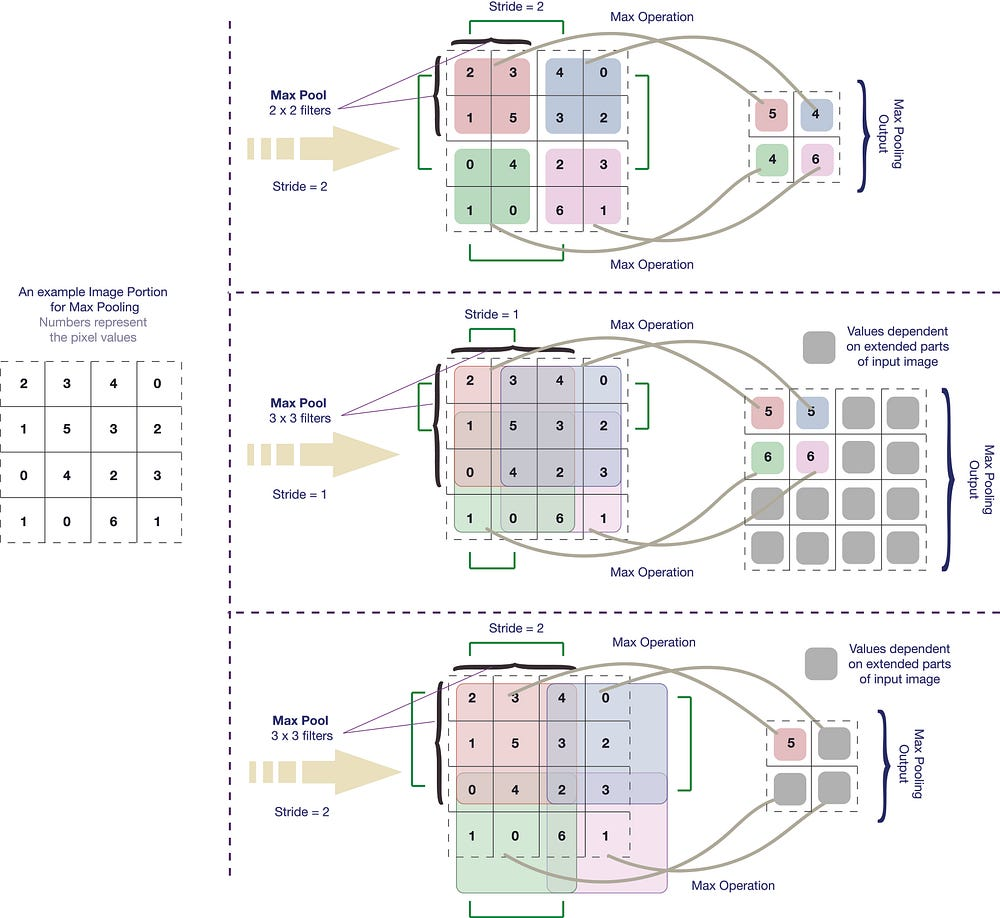The above image is self-explanatory. You can understand strides and max pool.

Max pooling is the application of a moving window across a 2D input space, where the maximum value within that window is the output. In the code, the size of the window is “pool_size = (2,2)”.

Dropout

When a large feedforward neural network is trained on a small training set, it typically performs poorly on held-out test data. This “overfitting” is greatly reduced by randomly omitting half of the feature detectors on each training case.

Improving neural networks by preventing co-adaptation of feature detectors. Each neuron learns to detect a feature that is generally helpful for producing the correct answer given the combinatorially large variety of internal contexts in which it must operate. I would recommend you to read the motivation section of this paper, it is interesting, engaging and helpful to understand the concept of dropout.

The term “dropout” refers to dropping out units (hidden and visible) in a neural network. By dropping a unit out, we mean temporarily removing it from the network, along with all its incoming and outgoing connections. The choice of which units to drop is random. In the simplest case, each unit is retained with a fixed probability p independent of other units, where p can be chosen using a validation set or can simply be set at 0.5, which seems to be close to optimal for a wide range of networks and tasks. For the input units, however, the optimal probability of retention is usually closer to 1 than to 0.5.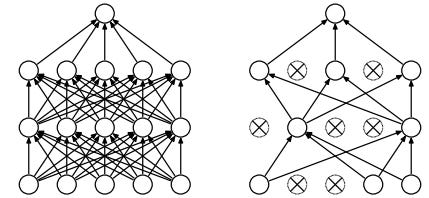Dropout Neural Net Model. Left: A standard neural net with 2 hidden layers. Right: An example of a thinned net produced by applying dropout to the network on the left. Crossed units have been dropped.

Dropout during testing and training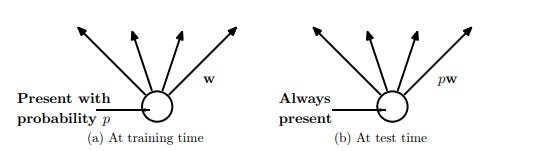Left: A unit at training time that is present with probability p and is connected to units in the next layer with weights w. Right: At test time, the unit is always present and the weights are multiplied by p. The output at test time is same as the expected output at training time.

Dropout roughly doubles the number of iterations required to converge. However, the training time for each epoch is less. Therefore it is suggested to use dropout with the batch normalization which speeds up the training process to a great extent.

In the same way from line 11 to 31, there are 6 convolution layers in this model. With 6 convolution layers, I mean to say

`{Convolution=>BatchNormalization=>Activation=>MaxPooling2D=> Dropout}`

The above representation is not a line of code or mathematical equation, it just a representation of the phrase “a convolution layer” which may have all of the other layers stacked with it like batchnorm, activation, Max pool, Dropout. With “may” I mean to say that we might interchange the position of the layers and also its parameter values. We can also add more layer. In all the convolution layers we have used a dropout value of 0.25. Which means 25% of the neurons will be randomly dropped while training and testing.

Line 32

A Flatten layer in Keras reshapes the tensor output coming from its previous layer that is the Max pool layer to a single column vector transforming the entire pooled feature map matrix into a single column which is then fed to the following layer neural network for further processing. In our case example code, the Fully connected layer is the layer following the flatten layer.

The fully connected layer is known as Dense layer. In the code model.add(Dense(512)) is the fully connected layer. The layer has 512 neurons. Each neuron in the flatten layer is connected to each neuron in the fully connected layer. The number 512 could be changed to any other number.

Finally, after several convolutional and max-pooling layers, the high-level reasoning in the neural network is done via fully connected layers. Neurons in a fully connected layer have connections to all activations in the previous layer, as seen in the regular non-convolution model. Convolution capture better representation of data and hence we don’t need to do feature engineering. After feature extraction we need to classify the data into various classes, this can be done using a fully connected (FC) neural network. The output from the convolutional layers represents high-level features in the data. While that output could be flattened and connected to the output layer, adding a fully-connected layer is a way of learning non-linear combinations of these features.

Here, the fully connected layer with 512 neurons is followed by batch normalization, activation layer (relu), and a dropout of 50%.

Line 41- On line number 41 you can see we are using another fully connected layer “model.add(Dense(classes))”, unlike the previous layer where we have a specified number of neuron number of neurons = 512 and claim that we can change the number of neurons to any number, this dense layer which is the last or output layer of the model will have (number of neurons == number of classes). Here, model.add(Dense(classes)) == model.add(Dense(3)).

Line 42- Unlike other layers which are followed by relu(activation function), this layer is followed by an activation function called softmax. Softmax activation is basically the normalized exponential probability of class observations represented as neuron activations. Used for multiclass classification. The sum of all the probabilities will be 1. I would highly recommend this article to read how softmax is implemented step by step.

Step — 5 Compile the model

Line 3- Here we are initializing the learning rate. Learning rate = 0.01. Learning rate is the rate with which the model should learn. The learning rate value is a small real value such as 0.1, 0.001 or 0.0001.The decision of how much our learning rate should depend on experimentation. Naive method for choosing learning rate is trying out a bunch of numbers and using the one that looks to work best, manually decreasing it over time when training doesn’t seem to improve the loss anymore. It tells how fast the weights must be learned.

Line 4-Here we define the number of epochs. Epoch is a unit. Here Epoch =75 means that model will be trained 75 times on every single training images. When every single image in a training dataset has at least undergone forward and backward propagation once then we say one epoch is completed.

Line 9 and, 10-Line 9 calls the Keras, stochastic gradient descent(SGD). SGD is an optimizer. It optimizes the model by reducing the loss calculated by the loss function (categorical cross entropy). Work of the loss function is to calculate the difference between the predicted and true values by the machine learning model which is getting trained. This difference is also called loss, the lesser it is the better it is. The behavior of loss helps the model to understand what must be done to optimize the model so that the loss can be reduced. We have used accuracy as the metrics here. The greater it is the better it is. Unlike the loss function, metrics do not play any role in optimization.

You can compile a network (model) as many times as you want. You need to compile the model if you wish to change the loss function, optimizer or matrices.

You need a compiled model to train (because training uses the loss function and the optimizer). But it’s not necessary to compile the model when testing the model on a new data.

“Categorical cross entropy” is the loss function used in the code. Work of the loss function is to calculate the difference between the predicted and expected values by the machine learning model which is getting trained. This difference is also called loss, the lesser it is the better it is. The behavior of loss helps the model to understand what must be done to optimize the model so that the loss can be reduced.

Cross-entropy is commonly used to quantify the difference between two probability distributions. Usually, the “true” distribution (the one that your machine learning algorithm is trying to match) is expressed in terms of a one-hot distribution.

For example, suppose for a specific training instance, the label is B (out of the possible labels A, B, and C). The one-hot distribution for this training instance is, therefore:

``Pr(Class A) Pr(Class B) Pr(Class C) 0.0 1.0 0.0``

You can interpret the above “true” distribution to mean that the training instance has 0% probability of being class A, 100% probability of being class B, and 0% probability of being class C.

Now, suppose your machine learning algorithm predicts the following probability distribution:

``Pr(Class A) Pr(Class B) Pr(Class C) 0.228 0.619 0.153``

How close is the predicted distribution to the true distribution? That is what the cross-entropy loss determines. Use this formula:

Where `p(x)` is the wanted probability, and `q(x)` the actual probability. The sum is over the three classes A, B, and C. In this case, the loss is 0.479 :

``H = - (0.0*ln(0.228) + 1.0*ln(0.619) + 0.0*ln(0.153)) = 0.479``

So that is how “wrong” or “far away” your prediction is from the true distribution.

Now lets, see what role does the metrics argument plays here. We have used accuracy as the metrics here. The greater it is the better it is. There are many reasons to have another parameter with loss function (Loss function works hand in hand with the optimizer to update the model, without the loss function the model can never be optimized as it will never know by what percentage it must optimize). On the other hand, metrics do not play any role in optimization. Please read this. It clearly states that it is just to measure the fitness of your model with respect to another maths (accuracy, recall, F1 score, cosine-distance), which you may want to use just for a reason to observe how these changes across epochs. Recall, accuracy, F1 score is used over accuracy. Accuracy can be misleading. For example, in a problem where there is a large number of imbalanced data for classes, a model can predict the value of the class which had huge train data for all successful predictions and achieves a high classification accuracy. It would be wrong to say that our model will match the prediction accuracy on test data as well because we know that there was an imbalance in the training data for different classes.

Step -6 Train the model

This is the switch to start the engine of all that we have read from the beginning. The first argument accepts the training data which is generated (data is augmented) on the fly while training. Performing data augmentation is a form of regularization, enabling our model to generalize better. However, applying data augmentation implies that our training data is no longer “static” — the data is constantly changing. Each new batch of data is randomly adjusted according to the parameters supplied to ImageDataGenerator.

Internally, Keras is using the following process when training a model with .fit_generator :

1. Keras calls the generator function supplied to .fit_generator (in this case, flow_from_directory).
2. The generator function yields a batch of size batch_size to the .fit_generator function.
3. The .fit_generator function accepts the batch of data, performs backpropagation (training), and updates the weights in our model.
4. This process is repeated until we have reached the desired number of epochs.

We must keep in mind that a Keras data generator is meant to loop infinitely — it should never return or exit.

Since the function is intended to loop infinitely, Keras has no ability to determine when one epoch stops and a new epoch begins.

Therefore, we compute the steps_per_epoch value as the total number of training data points divided by the batch size. Once Keras hits this step count it knows that it’s a new epoch.

``steps_per_epoch = TotalTrainingSamples / TrainingBatchSize``
``validation_steps = Totalvalidationimages / ValidationBatchSize``

Basically, the two variables are: how many batches per epoch you will yield.
This makes sure that at each epoch:

• You train exactly your entire training set
• You validate exactly your entire validation set
• Step — 7 Evaluate the model

Line 3 and 11 -While training we predict the results using Keras predict function.

It’s important that we evaluate on our testing data so we can obtain an unbiased (or as close to unbiased as possible) representation of how well our model is performing with data it has never been trained on.

To visualize our model prediction during training we can use a combination of the .predict method of the model along with the classification_report from scikit-learn

Line 15 to 26-Helps us to plot the performance of the model in every epoch. It helps us to access the history that at what point of the training(Epoch) the loss, accuracy was decreasing or increasing. This performance is saved in an image “training_performance.png”.

As I said, the positions of any layer in the convolution layer can be changed. You can see that there is a swift change in the second graph as compared to the first one. It is yet not a solved topic of where the batch normalization layer should be placed. Though the training was smooth but there was no major change in the validation accuracy.

Save your model so that you can use it next time to predict the results.

Test the model performance

You have trained the network with some accuracy. Now, you wish to use the model to predict the class of any test image. What do you do?

Create a python file with name predict.py

You will have to load three things first a test image (cat or dog or panda), trained model and the binarized label file.

Line 9,11,12 -Path of the test image, the trained model and the label binarized file is given.

Line 14 to 16-Test image data is converted from its original shape to a shape height =64, width =64, channels = 3

Line 19 to 23- The 64x64x3 image is converted to a numpy array of size (1,12288).

Line 27 and 28-The trained model and the label binarizer are loaded.

Line 32- Generates output predictions for the input test image.

Line 36–48- Helps you visualize the prediction result in the form of text and images.

When you run the predict.py code, the input is a cat image. The output would be.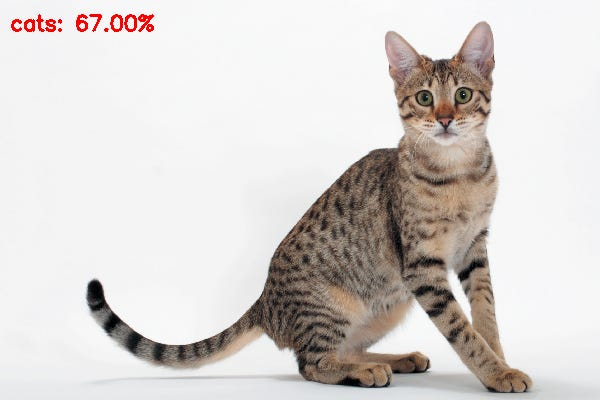Here you can see that the system has learned to classify the image correctly and with good accuracy. Even though the image has a lower body of the cat which is similar to the dog, there has been no misclassification unlike the model with fully connected layers. The credit goes to convolution layers.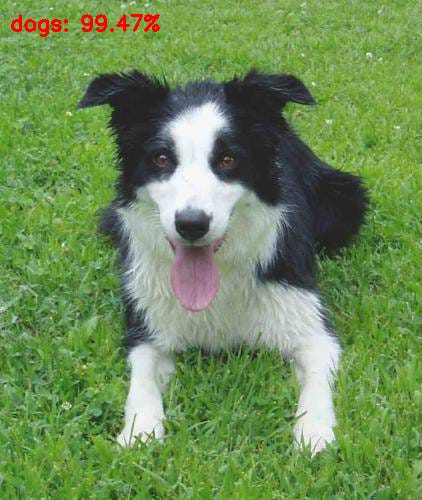The dog image is classified correctly with very high accuracy. If you test this image using the fully connected network you get a False negative where the image has a dog and it classifies the image as a panda.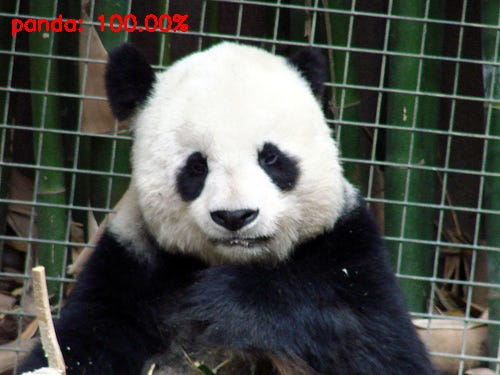This image is an example of true positive where a panda is correctly classified as a Panda.

Conclusion:

In this blog, we have learned to build our deep learning model using convolution neural network in Keras. Most importantly in this blog, we have trained and tested a multi-class classifier to classify dogs, cats, and pandas from scratch in Keras. We saw how the accuracies have increased and the misclassification has decreased. We also learned a new topic Batchnormalization. Each line of the code is explained in detail. We have learned why there is a need to move to convolution layer from the fully connected layer. I hope you enjoyed this part of AI Starter series. We also learned about hyperparameters. In the next blog, we will learn theories of learned parameters and memory optimization. Please give your kind feedback for this article, it will encourage and help me improve my work. Also, share it and follow to stay updated with such easy and detailed articles in the field of Machine learning, Deep learning, Computer Vision and Image processing.I am extremely happy to collaborate with Shubham Shrey a dexterous graphic designer. Special thanks for creating this thoughtful logo for my AI -Starter series.

Reference: